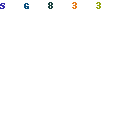Sine wave oscillatorI introduce the sine wave oscillator which used the operational amplifier in this page.
The Wien bridge sine wave oscillator to introduce is the oscillator which works in the oscillation by returning(positive feedback) the oscillation output to the input.
Because there are few parts, the Wien bridge oscillator is the often used circuit.
The point of this circuit is the negative feedback circuit to make the oscillation operation be stable. The circuit to be using this time changes the resistance value of the Field Effect-type Transistor(FET) at the d.c. voltage which rectified the oscillation output in the full wave and is making the oscillation operation be stable.
The sine wave oscillator is the oscillator which is difficult to make because the distortion of the oscillation signal occurs compared with the square wave oscillator, the triangular wave oscillator.

In case of C=C1=C2, R=R1=R2, the oscillation frequency can be calculated by the following formula.The example of the circuit which was made this time is shown below.
 f = 1/(2 x 3.14 x 0.01 x 10-6 x 15 x 103) = 1/(0.942 x 10-3) = 1.062 x 103 = 1,062 Hz

The frequency in the actual circuit was to 900 Hz be.

You can change the frequency when you change C1, C2, R1 and R2 of the circuit diagram. In the relation of the balance at the bridge, you had better make C1=C2, R1=R2.
Even if it is different little, it is possible to oscillate.

I am explaining the following contents in the page of the explanation.The sine wave is what?The principle of the oscillatorThe principle of the Wien bridge oscillation circuitThe amplitude control of the Wien bridge oscillationPattern drawingOperation explanation